# Civil Engineering - UPSC Civil Service Exam Questions

46.

Which one of the following expresses the height of rise or fall of a liquid in a capillary tube ?(w = Specific weight of the liquid α = Angle of contact of the liquid surface σ = Surface tension)

 A.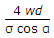B.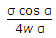C.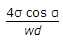D.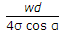Explanation:

No answer description available for this question. Let us discuss.

47.

Given that Plasticity Index (PI) of local soil = 15 and PI of sand = zero, for a desired PJ of 6, the percentage of sand in the mix should be

 A. 70 B. 60 C. 40 D. 30

Explanation:

No answer description available for this question. Let us discuss.

48.

Two closely-coiled springs A and B of the same material, same wire and same number of turns are subjected to an axial load of W. The mean diameter of spring A is half that of spring B. The ratio of deflection of spring B to that of spring A will be

 A. 8 B. 4 C. 1/4 D. 1/8

Explanation:

No answer description available for this question. Let us discuss.

49.

A column ABCD (2y1 x 2y2) of rectangular section carries a load P at Z having the co-ordinates (x, y) as shown in the given figure.
If the compressive streses are taken as positive and area A = 2y1 x 2y2 = 4y1 y2 and the moment of inertia about x and y axis being Ixx and Iyy respectively, then the stress at the corner D is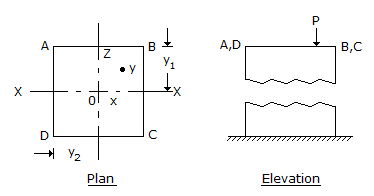A.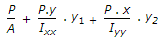B.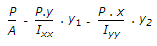C.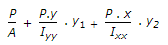D.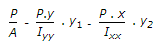Explanation:

No answer description available for this question. Let us discuss.

50.

What are leaping weirs ?

 A. sewage flow measuring devices B. storm regulators C. velocity control devices D. sewer out falls